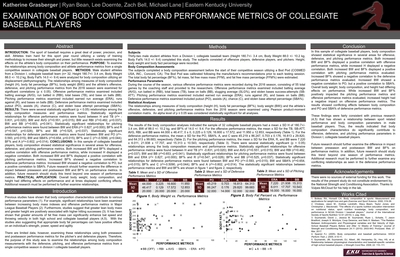## University Presentation Showcase: Undergraduate Poster Gallery

#### PreviewSpring 2017

Chemistry

Chemistry

Michael T. Lane

#### Mentor Department

Exercise and Sport Science

#### Abstract

METHODS: Male student-athletes from a DI, collegiate baseball team (n= 32, Height 180.7+/-3.4 cm, Body Weight 88.0+/-10.2 kg, Body Fat% 14.0+/-9.4) were analyzed for body composition utilizing air displacement plethysmography. The relationships among measures of body composition (height (H), body fat percentage (BF%), body weight (BW)) and athlete’s offensive, defensive, and pitchers’ performance metrics from the 2016 season were examined for significant correlations (p ≤ 0.05). Offensive performance metrics: batting average (AVG), run batted in (RBI), total bases (TB), base on balls (BB), slugging average (SLG%), stolen bases success-attempts (SB-ATT). Pitching performance metrics: earned run average (ERA), runs against (R), bases on balls (BB). Defensive performance metrics: putout (PO), assists (A), chance (C), stolen base attempt percentage (SBA%). RESULTS: There were several statistically significant (p < 0.05) relationships. Relationships for offensive performance metrics were found between H and TB (r2= -0.831, p=0.020); BF% and SB-ATT (r2= 0.769, p=0.035); BW and AVG (r2=0.551, p=0.015), RBI (r2=0.482, p=0.037),BB (r2=0.653, p=0.041). Relationships for pitcher’s performance metrics were found between BW and ERA (r2= 0.827, p=0.000); BF% and R (r2=0.547, p=0.028), BB (r2=0.525, p=0.037). Relationships for defensive performance metrics were found between BW and PO (r2=-0.593, p=0.015), SBA% (r2=0.654, p=0.015); BF% and C (r2=-0.5090, p=0.044), A (r2=-0.602, p=0.014). CONCLUSIONS: In this sample, body composition showed statistical significance in several areas for offensive, defensive, and pitchers’ performance metrics. Future research should further examine the difference in impact between preseason and postseason body composition on performance metrics and study this trend beyond one season.

COinS